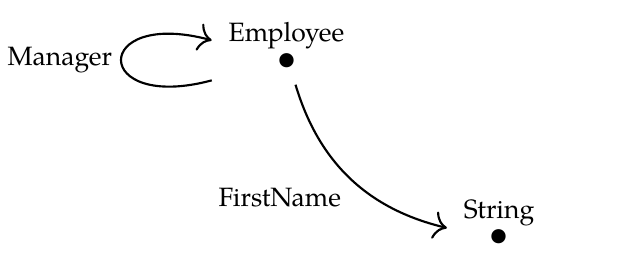Okay, so continuing on... let \$$\mathcal{D}\$$ be the free category on this graph:and let \$$\mathcal{C}\$$ be the free category on this graph:We got an obvious 'inclusion' functor \$$G : \mathcal{D} \to \mathcal{C}\$$. Now, let's try to think of this as a 'forgetful' functor. There's no technical definition of a forgetful functor, so this is just a matter of _attitude_. We need to imagine the objects of \$$\mathcal{C}\$$ and \$$\mathcal{D}\$$ as 'mathematical objects' of some kind. Their names fight against this way of thinking! That's why this problem is tough. But try.

The functor \$$G\\$$ is full, faithful, but not essentially surjective. This basically says that \$$\mathcal{C}\$$ has more objects than \$$\mathcal{D}\$$, but if you have two objects of \$$\mathcal{C}\$$ that came from objects in \$$\mathcal{D}\$$, the morphisms between them in \$$\mathcal{D}\$$ are the same as the morphisms between them in \$$\mathcal{C}\$$

A great mathematical example of this sort of situation is where\$$\mathcal{D} \$$ is the category of abelian groups and \$$\mathcal{C}\$$ is the category of groups. Here the forgetful functor \$$G : \mathcal{D} \to \mathcal{C}\$$ forgets the property of being abelian.

In _our_ example, therefore, \$$G\$$ is forgetting the property of being "not \$$\textbf{Department}\$$". That's a property that all objects of \$$\mathcal{D}\$$ have, but not all objects of \$$\mathcal{C}\$$.

I know this sounds weird. But it's just a psychological thing. If we regard the objects of \$$\mathcal{D}\$$ as mathematical gadgets of some sort, and the objects of \$$\mathcal{C}\$$ as mathematical gadgets with some extra property, then it's not so bad.